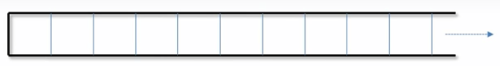# C++ STL Vector Container – std::vector

Here you will learn about C++ STL Vector Container i.e. std::vector and various functions applicable on it.

Vector, as we discussed earlier vector is dynamic array that grows in one direction.Also Read: C++ STL Array Container – std::array

## C++ STL Vector

Vectors are dynamic arrays. When we insert a new element or delete an element from vector, it has ability to resize itself automatically. Vector elements also stored in contiguous memory locations, so that they can traverse and accessed by iterators. Inserting and deleting at end takes constant , O(1) time. By using vectors we can avoid array_index_outof_bound exceptions.

### Syntax of STL Vector

vector <data_type> vectorName;

### Functions Used with Vector Container

push_back(): This is used to insert element into vector. It inserts element at the end of the vector.

[] operator: This operator returns the reference of the element positioned at index where we access like vectorName[index_position].

at(): This operator also useful to access the element at particular position.

front(): This returns the reference to the first element of the vector.

back(): This returns the reference to the last element of the vector.

Example program to show above mentioned functions:

Output

0 1 2 3 4 5
0
5

size(): It gives the number of elements present in the vector.

max_size(): It gives the maximum number of elements that a vector can hold.

capacity(): We said that, vector is dynamic array which grow by inserting elements. When we declare it system allocates some space to it. Particular number of elements it can hold. If we insert more than that elements, system allocates some more space for it (at new location by free-up old space).

Capacity() returns how many items can be fit in the vector before it is “full”. Once full, adding new items will result in a new, larger block of memory being allocated and the existing items being copied to it.

resize(): It resize the vector, restrict to contain only some number of elements.

empty(): This is Boolean function. Returns whether the vector is empty or not.

Example Program to show above functions:

Output

size of the vector is 5
Maximum size is 4611686018427387903
Capacity of the vector is 8
vector is empty

Vector with Iterator

begin(): Returns an iterator pointing to the first element of the vector.

end(): Returns an iterator pointing to the present last element of the vector.

rbegin(): Returns a reverse iterator pointing to the last element in the vector.  Used to move from last to first

rend(): Returns a reverse iterator pointing to the first element in the vector.

Example program:

Output

elements in the vector from start to end 0 1 2 3 4
elements in the vector from end to start 4 3 2 1 0

assign(): Assign new content to vector and resize.

pop_back(): This removes the element at the end of the vector. So that, size of the vector also reduced by 1.

insert(iterator, element): This inserts the element in vector before the position pointed by iterator. This insert method can be overloaded by third variable count. This says how many times the element to be inserted before the pointed position.

Example program to show above methods:

Output

Vector1 elements are
100 100 100 100
Vector2 elements are
100 100 100
new value inserted into vector2. Last element is 10
after pop_back operation last element of vector2 is 100
Now first element of vec3 is 20

erase(): Removes the element pointed by the iterator position. This erase method can be overloaded with extra iterator that specifies the range to be removed.

Example program:

Output

first element before erasing is 100
first element after erasing is 0
vector is empty

swap( vector1, vector2): This swaps the all elements of vector1 to vector2 and vector2 to vector1.

clear(): This removes the all elements of the vector.

Example program:

Output

Vector1 elements before swapping are
1 2 3 4 5
Vector2 elements before swapping are
11 12 13 14 15
Vector1 elements after swapping are
11 12 13 14 15
Vector2 elements after swapping are
1 2 3 4 5
vector is empty

Comment below if you have queries or found any information incorrect in above tutorial for C++ stl vector container.

### 1 thought on “C++ STL Vector Container – std::vector”

1.Very good explaination was searching for a site like this to learn STL. Found it at last. Keep up the great work.